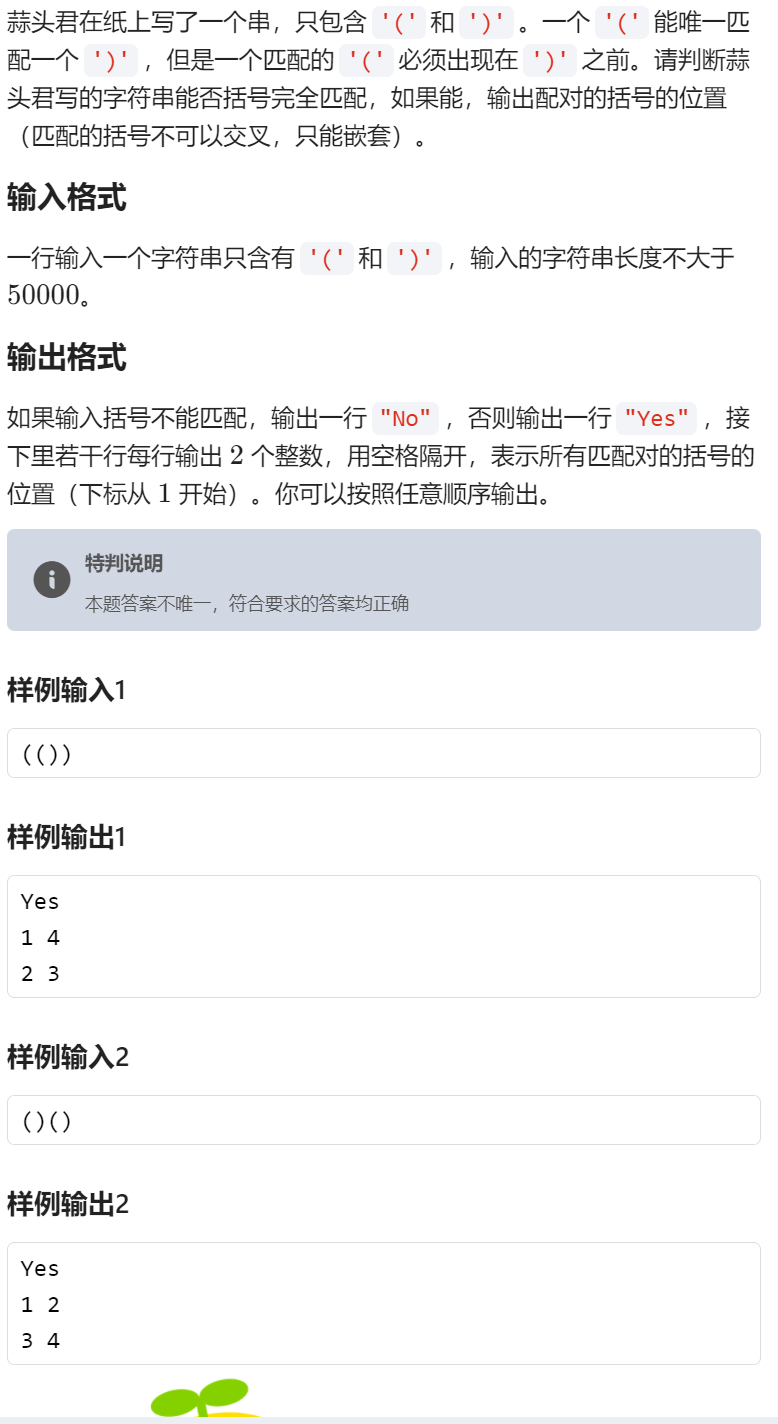括号匹配 （栈

2021/7/21 20:19:21 浏览：

输出格式#include<stdio.h>
struct kuohao{
char kh;
int dirt;
}s,st;
int main(){
int top=-1;
int i=0;
int dirction;
int cnt=0;
do{
scanf("%c",&s[i].kh);
s[i].dirt=i+1;
i++;
}while(s[i-1].kh!='\0');

for(i=0;s[i].kh!='\0';i++){
if(s[i].kh=='(') {st[++top]=s[i];}
else if(s[i].kh==')'&&top!=-1) {
dirction[cnt]=st[top].dirt;
dirction[cnt]=s[i].dirt;
cnt++;
top--;}
else if(s[i].kh==')'&&top==-1) {printf("No");return 0;}

}
if(top!=-1) { printf("No");return 0;}
printf("Yes\n");
for(i=0;i<cnt;i++)printf("%d %d\n",dirction[i],dirction[i]);
return 0;
}